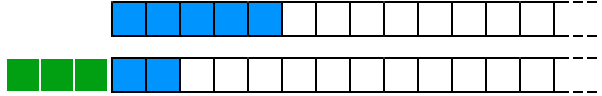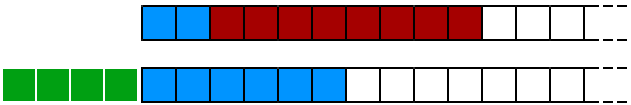Previously, I discussed the Huffman compression algorithm as implemented by JPEG, and a mechanism by which JPEG encoders pick the substitution values used for the compression. The image itself, in a baseline entropy-coded JPEG file, is stored in one "scan" as a stream of Huffman codes; the codes are of a variable length, and are not necessarily at even byte boundaries.

For this reason, it's a requirement that some kind of queue be introduced between the bytes of the file, and the values as seen by the JPEG decoder: this allows bits to be pushed onto the queue from the bytes of the file, and pulled out in varying amounts by the decoder. A single routine can thus be made the central routing point for any requests for variable-width symbols.

A queue of things, when used in this fashion, generally has two modes of operation: either there are enough things in the queue for the request to deal with, or additional reading has to be done before there are enough things in the queue to handle the request. In the case of a bit-level queue for reading from a file, as we need here, the number of bits requested is the determining factor. In the first example below, a queue holding five bits is asked to return the first three, and is able to handle the request without a problem.Figure 1: Request handling with sufficient content in the queue

If a request for four bits then comes in, the queue doesn't contain enough bits to handle the request, and must first fetch another full byte from the JPEG file (shown here in red).Figure 2: Request handling when the queue is empty

### Implementing the bit queue

A complication arises when trying to code an implementation of the bit queue as shown above: the output end of the queue is at the left, and if one contiguous value is used to store the queue, the left is the most-significant end with the highest-value bits. If we demarcate the queue as being 32 positions long, and define one 32-bit value as holding its entire contents, the above scenarios break down as follows:

Pulling three bits
• The request is shorter than the queue length of 5, no reading from file happens.
• The output value is the top three bits of the queue, shifted down (32 - 3) bits to become the bottom three bits of an output value;
• The queue is shifted left three bits, and left with length of 2.
Pulling another four bits
• The request is longer than the queue length of 2: a byte is read in, and shifted up (24 - 2) bits to join the queue;
• The queue is now 10 bits long, no more bytes need reading;
• The output value is the top four bits of the queue, shifted down (32 - 4);
• The queue is shifted left four bits, and left with length of 6.

At all times during the queue's operation, the next available bits are at the high-value end of the contiguous queue variable. An implementation of this may look like the following.

#### jpeg.h: Definition of bit-resolution file reader

class JPEG { private: // Read a number of bits from file u16 readBits(int); };

u16 JPEG::readBits(int len) { // The number of bits left in the queue, and the value represented static int queueLen = 0; static u32 queueVal = 0; u8 readByte; u16 output; if (len > queueLen) { do { // Read a byte in, shift it up to join the queue readByte = fgetc(fp); queueVal = queueVal | (readByte << (24 - queueLen)); queueLen += 8; } while (len > queueLen); } // Shift the requested number of bytes down to the other end output = ((queueVal >> (32 - len)) & ((1 << len) - 1)); queueLen -= len; queueVal <<= len; return output; }

### The JPEG SOF segment

As mentioned in the previous part of this series, a JPEG file can define up to 32 Huffman code tables, each in their own `DHT` segment. A JPEG file holds the data corresponding to the image itself in a "frame", denoted by a "Start of Frame" segment header. The SOF header contains a part of the information required to decode the frame, and is structured according to the following table.

FieldValueSize (bytes)
Precision (the number of pixels in a JPEG block)81
Image heightUp to 655352
Image widthUp to 655352
ComponentsNumber of colour components1
For each component (in a YUV-colour file, three)
IDIdentifier for later use1
Sampling resolutionFor later examination1
Quantisation tableFor later examination1
Table 1: Structure of the SOF header

As can be seen in the above table, some of the fields involve operations that we have not yet examined (the sampling resolution and the quantisation table for each component). For the purposes of completing the SOF segment handler, we can hold onto this information for later use.

A "frame" consists of the SOF header, and a number of "scans"; as the name implies, each scan is a pass over the full rectangle of the image. In an interlaced JPEG file for example, multiple scans would be present for the single frame, each of them having more resolution than the last; in a progressive JPEG file, there is just one scan containing all the information for the image. Since this series is concerned with building a decoder for progressive JPEG files, we'll focus on dealing with a single scan in the frame.

It turns out that the information required by Huffman decoding, in particular which of the `DHT` tables to use, is defined by each scan in the frame instead of by the frame itself. We'll look at the scan-level information in more detail in the next part of this series; for now, it's sufficient to decouple our representation of a colour component in the image from the `SOF` data, and define the exact metadata for a component later.

### Structure definitions

With the information detailing the structure of an `SOF` header, it becomes relatively simple to build a segment parser to plug into our existing code. The only complication arises from the fact that multi-byte values in JPEG are stored in big-endian format, which may not necessarily be the host format for large integers. It's useful to have a set of definitions for transparently handling big-endian values, which is presented below.

#### byteswap.h: Big-endian value handling macros

/** * Let's Build a JPEG Decoder * Big-endian value handling macros * Imran Nazar, May 2013 */ #ifndef __BYTESWAP_H_ #define __BYTESWAP_H_ #if __SYS_BIG_ENDIAN == 1 # define htoms(x) (x) # define htoml(x) (x) # define mtohs(x) (x) # define mtohl(x) (x) #else # define htoms(x) (((x)>>8)|((x)<<8)) # define htoml(x) (((x)<<24)|(((x)&0xFF00)<<8)|(((x)&0xFF0000)>>8)|((x)>>24)) # define mtohs(x) (((x)>>8)|((x)<<8)) # define mtohl(x) (((x)<<24)|(((x)&0xFF00)<<8)|(((x)&0xFF0000)>>8)|((x)>>24)) #endif #endif//__BYTESWAP_H_

// Prevent padding bytes from creeping into structures #define PACKED __attribute__((packed)) class JPEG { private: // Information in the SOF header struct PACKED { u8 precision; u16 height; u16 width; u8 component_count; } sofHead; typedef struct PACKED { u8 id; u8 sampling; u8 q_table; } sofComponent; // Internal information about a colour component typedef struct PACKED { u8 id; // There is likely to be more data here... } Component; // The set of colour components in the image std::vector components; // The SOF segment handler int SOF(); };

#### jpeg.cpp: Passing control to the segment handler

int JPEG::parseSeg() { ... switch (id) { // The SOF segment defines the components and resolution // of the JPEG frame for a baseline Huffman-coded image case 0xFFC0: size = READ_WORD() - 2; if (SOF() != size) { printf("Unexpected end of SOF segment\n"); return JPEG_SEG_ERR; } break; ... } return JPEG_SEG_OK; }